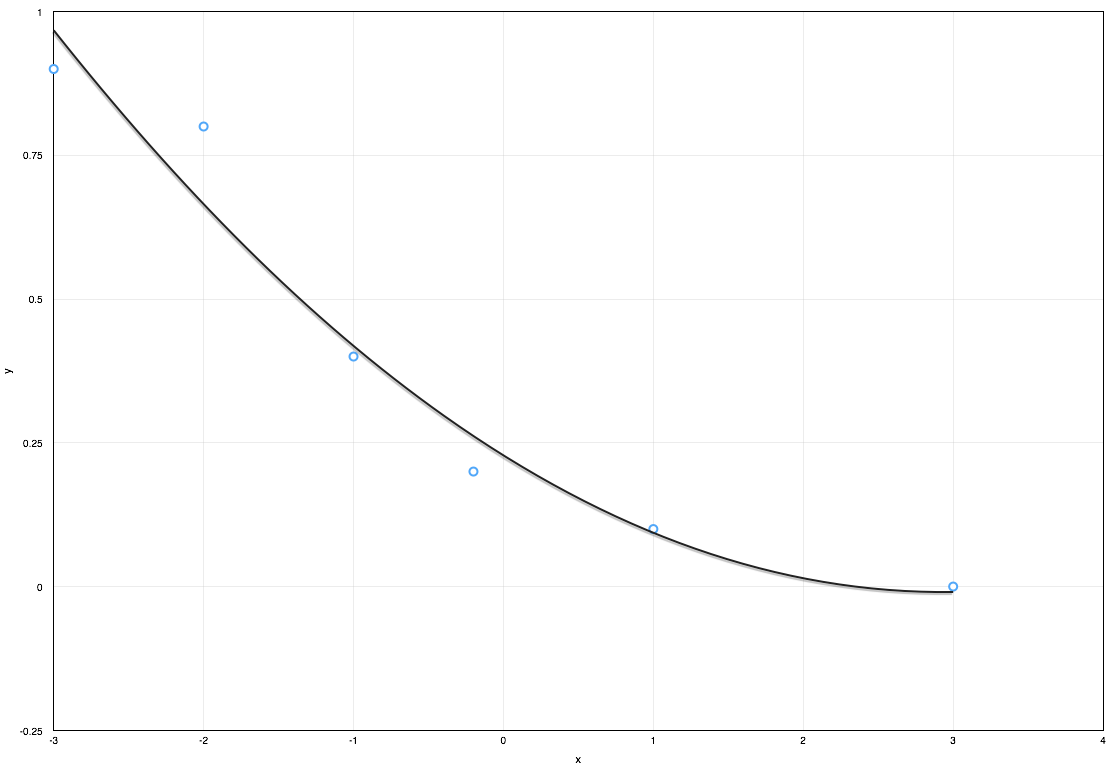f
Neutrium

# Linear Algebra

Fitting of a Polynomial using Least Squares MethodApproximating a dataset using a polynomial equation is useful when conducting engineering calculations as it allows results to be quickly updated when inputs change without the need for manual lookup of the dataset. The most common method to generate a polynomial equation from a given data set is the least squares method. This article demonstrates how to generate a polynomial curve fit using the least squares method.